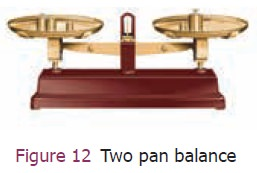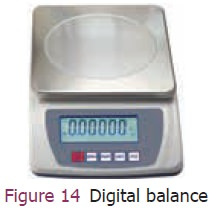Home | | Science 9th std | Measuring Mass

# Measuring Mass

We commonly use the term ‘weight’ which is actually the ‘mass’. Many things are measured in terms of ‘mass’ in the commercial world.

Measuring Mass

We commonly use the term ‘weight’ which is actually the ‘mass’. Many things are measured in terms of ‘mass’ in the commercial world. The SI unit of mass is kilogram. In any case, the units are based on the items purchased. For example, we buy gold in gram or milligram, medicines in milligram, provisions in gram and kilogram and express cargo in tonnes.

Can we use the same instrument for measuring the above listed items? Different measuring devices have to be used for items of smaller and larger masses. In this section we will study about some of the instruments used for measuring mass.

## Common (beam) balance

A beam balance compares the sample mass with a standard reference mass. (Standard reference masses are 5g, 10g, 20g, 50g, 100g, 200g, 500g, 1kg, 2kg, 5kg). is balance can measure mass accurately up to 5g (Figure 11).## Two pan balance

This type of balance is commonly used in provision and grocery shops (Figure 12). is balance compares the sample mass with the standard reference mass. The pans rest on top of the beam and can be conveniently placed on a table top. is balance can measure mass accurately upto 5 g.## Physical balance

This balance is used in labs and is similar to the beam balance but it is a lot more sensitive and can measure mass of an object correct to a milligram (Figure 13).

The standard reference masses used in this physical balance are 10 mg, 20 mg, 50 mg, 100 mg, 200 mg, 500 mg, 1 g, 2g, 5 g, 10 g, 20 g, 50 g, 100g, and 200 g.## Digital balance

Nowadays for accurate measurements digital balances are used, which measures mass accurately even up to a few milligrams, the least value being 10 mg (Figure 14). is electrical device is easy to handle and commonly used in jewellery shops and labs.## Spring balance

This balance helps us to find the weight of an object. It consists of a spring fixed at one end and a hook attached to a rod at the other end. It works by ‘Hooke’s law’ which explains that the addition of weight produces a proportional increase in the length of the spring (Figure 15). A pointer is attached to the rod which slides over a graduated scale on the right. The spring extends according to the weight attached to the hook and the pointer reads the weight of the object on the scale.Solve – The mass of 40 apples in a box is 10 kg. (i) Find the mass of a dozen of them (ii) Express the mass of one apple in gram.

## 1. Difference between massand weight

Mass (m) is the quantity of matter contained in a body. Weight (w) is the normal force (N) exerted by the surface on the body to balance against gravitational pull on the object. In the case of spring scale the tension in the spring balances the gravitational pull on the object. When the man is standing on the surface of the earth or floor, the surface exerts a normal force on the body which is equivalent to gravitational force. The gravitational force acting on the object is given by ‘mg’. Here m is mass of the object and ‘g’ is acceleration due to gravity.

If a man has a mass 50 kg on the earth, then what is his weight?

Weight (w) = mg

Mass of a man = 50 kg

His weight = 50 × 9.8

w = 490 newtonThe pull of gravity on the Moon is 1/6 times weaker than that on the Earth. is causes the weight of the object on the Moon to be less than that on the Earth.

Acceleration due to gravity on the Moon = 1.63m/s2

If the mass of a man is 70 kg then his weight on the Earth is 686 N and on the Moon is 114 N. But his mass is still 70 kg on the Moon.

Tags : Measuring Instruments , 9th Science : Measurement and Measuring Instruments
Study Material, Lecturing Notes, Assignment, Reference, Wiki description explanation, brief detail
9th Science : Measurement and Measuring Instruments : Measuring Mass | Measuring Instruments